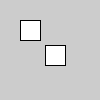# Py5Graphics.begin_draw()#

Sets the default properties for a `Py5Graphics` object.

## Examples#```def setup():
py5.size(100, 100, py5.P2D)

g = py5.create_graphics(60, 60, py5.P2D)
g.begin_draw()
g.translate(30, 30)
g.begin_shape()
g.vertex(-10, -10)
g.vertex(10, -10)
g.vertex(10, 10)
g.vertex(-10, 10)
g.end_shape(py5.CLOSE)
g.end_draw()

py5.image(g, 0, 0)
py5.image(g, 25, 25)
``````def setup():
py5.size(100, 100, py5.P2D)

g = py5.create_graphics(60, 60, py5.P2D)
with g.begin_draw():
g.translate(30, 30)
with g.begin_closed_shape():
g.vertex(-10, -10)
g.vertex(10, -10)
g.vertex(10, 10)
g.vertex(-10, 10)

py5.image(g, 0, 0)
py5.image(g, 25, 25)
```

## Description#

Sets the default properties for a `Py5Graphics` object. It should be called before anything is drawn into the object. After the drawing commands have concluded, call Py5Graphics.end_draw() to finalize the `Py5Graphics` object.

This method can be used as a context manager to ensure that Py5Graphics.end_draw() always gets called, as shown in the second example.

Underlying Processing method: PGraphics.beginDraw

## Signatures#

```begin_draw() -> None
```

Updated on September 01, 2022 16:36:02pm UTC